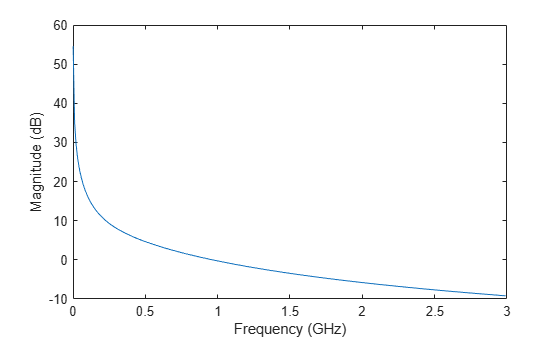# rfmodel.rational

Perform rational fit using pole-residue representation of the component

## Description

Use the `rational` class to represent RF components using a rational function object of the form:

`$F\left(s\right)=\left(\sum _{k=1}^{n}\frac{{C}_{k}}{s-{A}_{k}}+D\right){e}^{-s\tau }\begin{array}{cc},& s=j2\pi f\end{array}$`

There are two ways to construct an rational function object:

• You can fit a rational function object to the component data using the `rationalfit` function.

• You can use the `rfmodel.rational` constructor to specify the pole-residue representation of the component directly.

## Creation

### Syntax

``h = rfmodel.rational``
``````h = rfmodel.rational('Property1',value1,'Property2',value2,...)``````

### Description

example

````h = rfmodel.rational` returns a rational function object whose properties are set to their default values.```
``````h = rfmodel.rational('Property1',value1,'Property2',value2,...)``` sets properties using one or more name-value pairs. You can specify multiple name-value pairs. Enclose each property name in a quote```

## Properties

expand all

Poles of rational function object, specified as a complex vector in radians/second. The property length is shown in:

`$F\left(s\right)=\left(\sum _{k=1}^{n}\frac{{C}_{k}}{s-{A}_{k}}+D\right){e}^{-s\tau }\begin{array}{cc},& s=j2\pi f\end{array}$`

where, n must be equal to the length of the vector you provide for `'C'`. n is the number of poles in the rational function object. By default, this property is empty.

Data Types: `double`

Residues of the rational function object, specified as a complex vector in radians/second. The property length is shown in

`$F\left(s\right)=\left(\sum _{k=1}^{n}\frac{{C}_{k}}{s-{A}_{k}}+D\right){e}^{-s\tau }\begin{array}{cc},& s=j2\pi f\end{array}$`

as `n`, must be equal to the length of the vector you provide for `'A'`. `n` is the number of residues in the rational function object. By default, this property is empty.

Data Types: `double`

Frequency response offset, specified as a scalar. The default value is `0`.

Data Types: `double`

Frequency response time delay, specified as a scalar. The default value is `0`.

Data Types: `double`

Object name, specified as a `1-by-N` character array. This is a read-only property.

Data Types: `char`

## Object Functions

 `timeresp` Time response for rational objects `stepresp` Step-signal response for rational object and `rationalfit` function object `freqresp` Frequency response of rational object and `rationalfit` function object `impulse` Impulse response for rational function object `ispassive` Return true if `rationalfit` output is passive at all frequencies `makepassive` Enforce passivity of `rationalfit` output or a rational object `passivity` Plot passivity of N-by-N `rationalfit` function output `pwlresp` Calculate time response of piecewise linear input signal `generateSPICE` Generate SPICE file from `rationalfit` of S-parameters `writeva` Generate Verilog-A description of `rational` object `abcd` Construct state-space matrices from `rational` object `zpk` Compute zeros, poles, and gain of rational object

## Examples

collapse all

Fit a rational function to data from an `rfdata.data` object.

```S = sparameters('defaultbandpass.s2p'); freq = S.Frequencies; data = rfparam(S,2,1); fit = rationalfit(freq,data)```
```fit = rfmodel.rational with properties: A: [10x1 double] C: [10x1 double] D: 0 Delay: 0 Name: 'Rational Function' ```

Construct a rational function object, `rat`, with poles at -4 Mrad/s, -3 Grad/s,and -5 Grad/s and residues of 600 Mrad/s,2 Grad/s and 4 Grad/s.

`rat=rfmodel.rational('A',[-5e9,-3e9,-4e6],'C',[6e8,2e9,4e9]);`

Perform frequency-domain analysis from 1.0 MHz to 3.0 GHz.

`f = [1e6:1.0e7:3e9];`

Plot the resulting frequency response in decibels on the X-Y plane.

```[resp,freq]=freqresp(rat,f); figure plot(freq/1e9,20*log10(abs(resp))); xlabel('Frequency (GHz)') ylabel('Magnitude (dB)')```Read a file named `passive.s2p `and fit the 2-by-2 S-parameters. Generate a SPICE file of these S-parameters.

```S = sparameters('passive.s2p'); fit = rationalfit(S); generateSPICE(fit,'passive.ckt')```

The circuit is saved in your current folder.

## Version History

Introduced in R2009a